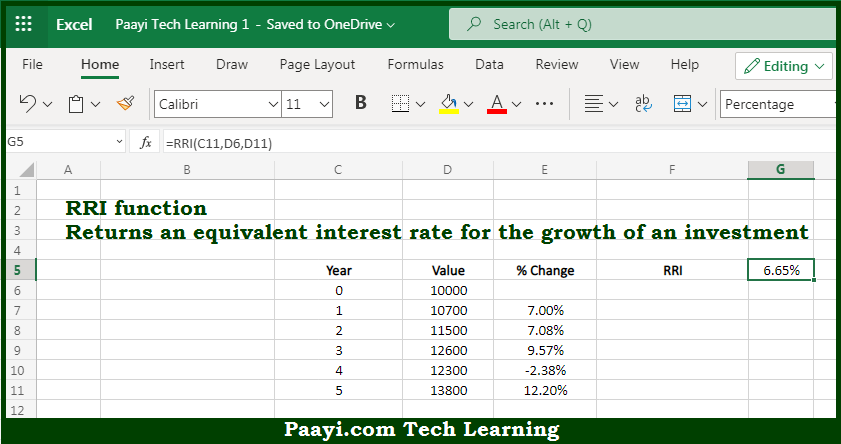# Learn How to Use Microsoft Excel RRI Function

Written by | 0 Comments | 618 Views

In this article, you will learn how to use the Microsoft Excel RRI function and its prime function in Microsoft Excel. You will also get to know the Microsoft Excel RRI function return value and syntax with the help of some examples.

Microsoft Excel RRI Function

The main purpose of the Microsoft Excel RRI function is to get the equivalent interest rate for the growth. That implies, with the help of the RRI function you can able to return the equivalent interest rate for the growth of the investment you have made. It should be noted that the RRI function can be used to calculate the Compound Annual Growth Rate (CAGR) in Microsoft Excel. So, with the help of the RRI function, you can able to get the equivalent interest rate for the growth.

Return Value of RRI Function

The return value will be the calculated interest rate.

Syntax of RRI Function

=RRI(nper, pv, fv)

Where the arguments:

• nper: This is the number of periods.
• pv: This is the present value of an investment.
• fv: This is the future value of an investment.

How to Use Microsoft Excel RRI Function?So we know that Microsoft Excel RRI function you can able to get the equivalent interest rate for the growth. That implies, with the help of the RRI function you can able to return the equivalent interest rate for the growth of the investment you have made. It should be noted that the RRI function can be used to calculate the Compound Annual Growth Rate (CAGR) in Microsoft Excel. So, with the help of the RRI function, you can able to get the equivalent interest rate for the growth.